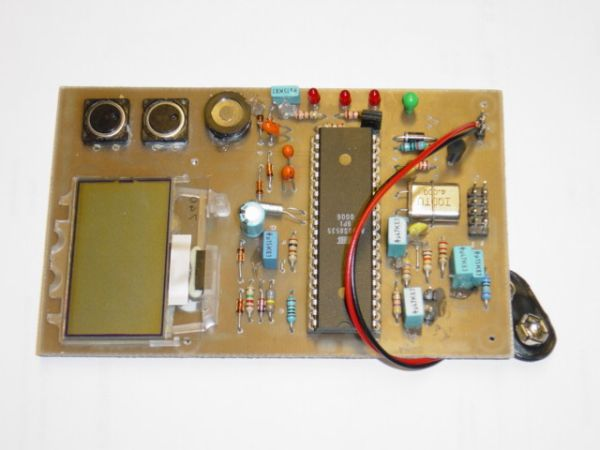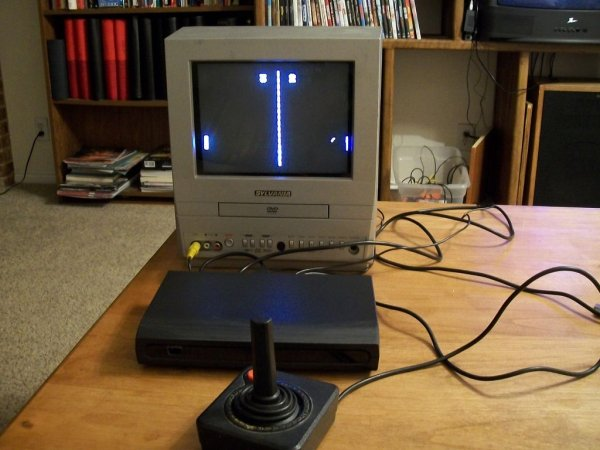# Bullit Accelerometer – Accelerometer with microcontroller and display

The accelerometer can measure acceleration in two axis using Analog Devices ADXL202. +-2g can be measured in each axis. Look herefor datasheets and application notes.

## Features

The accelerometer can measure acceleration and time. Using the time and the acceleration it can calculate and display:

• Time (s)
• Average acceleration (m/s2)
• Instantanous acceleration (min/max) (m/s2)
• Speed (m/s & km/h)
• Distance (m & km)

The mass of the object can also be input, the accelerometer can then also display:

• Instantenous Force (N)
• Average Force (N)
• Instantenous Power (kW and HP) * .

See the User Manual.

## HardwareThe sensor is the Analog Devices ADXL202.

CPU is an ATMEL AT90S8535 running at 4Mhz. A 32768 Hz crystal is connected to the real time clock timer inputs (TIMER2) and is the time base for the accelerometer. This allows a ceramic resonator to be used instead of a crystal if desired. A ceramic resonator should provide even lower power consumption. Power to all subcomponents like display and accelerometer senser are controlled by the CPU so that they can be turned off to save power.

READ  Large area industrial crystalline silicon n-PERT solar cell with 22 percent efficiency

The ADXL is connected to the Input Capture Pin (ICP) and the analog comparator. Rset of the ADXL that controls the period time is set so that there is approximatly 20000 ticks to one period.

The LCD display have 3 rows and is connected to the CPU using two data pins. The LCD uses I2C protocol and uses a variant of this driver circuit. A negative voltage charge pump is driven by the CPU to generate the bias voltage needed by the display. By measuring the bias voltage voltage with the ADC the CPU can regulate the negative voltage. A voltage divider between the negative lcd bias and the battery makes the voltage in a range to be measured.

Vref to the ADC is connected directly to the battery. One of the ADC inputs is connected to a reference voltage from a zener diode. The zener is driven by one of the pins from the CPU. This minimises the current consumption by just turning on the reference voltage when Vbatt need to be calculated from measurements of the zener voltage.

#### This Post / Project can also be found using search terms:

• akcelerometr akcelerometr